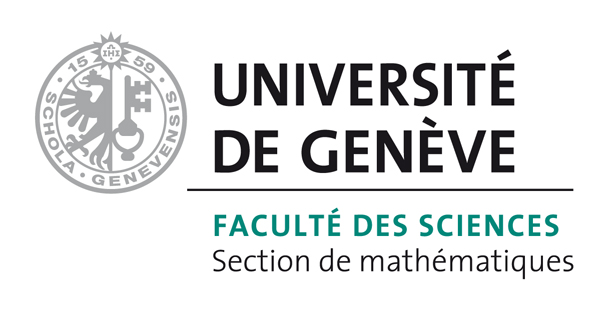# Bart Vandereycken## Riemannian multigrid line search for low-rank problems

by ,

#### Abstract:

Large-scale optimization problems arising from the discretization of problems involving PDEs sometimes admit solutions that can be well approximated by low-rank matrices. In this paper, we will exploit this low-rank approximation property by solving the optimization problem directly over the set of low-rank matrices. In particular, we introduce a new multilevel algorithm, where the optimization variable is constrained to the Riemannian manifold of fixed-rank matrices. In contrast to most other multilevel algorithms, where the rank is chosen adaptively on each level in order to control the perturbation due to the low-rank truncation, we can keep the ranks (and thus the computational complexity) fixed throughout the iterations. Furthermore, classical implementations of line searches based on Wolfe conditions enable computing a solution where the numerical accuracy is limited to about the square root of the machine epsilon. Here, we propose an extension to Riemannian manifolds of the line search of Hager and Zhang, which uses approximate Wolfe conditions that enable computing a solution on the order of the machine epsilon. Numerical experiments demonstrate the computational efficiency of the proposed framework.

#### Reference:

M. Sutti, B. Vandereycken, "Riemannian multigrid line search for low-rank problems", In SIAM J. Sci. Comput., pp. A1803-A1831, 2021.

#### Bibtex Entry:

@article{Sutti_V:2021,
title = {Riemannian multigrid line search for low-rank problems},
author = {Sutti, M. and Vandereycken, B.},
year = {2021},
pages = {A1803-A1831},
doi = {10.1137/20M1337430},
abstract = {Large-scale optimization problems arising from the discretization of problems involving PDEs sometimes admit solutions that can be well approximated by low-rank matrices. In this paper, we will exploit this low-rank approximation property by solving the optimization problem directly over the set of low-rank matrices. In particular, we introduce a new multilevel algorithm, where the optimization variable is constrained to the Riemannian manifold of fixed-rank matrices. In contrast to most other multilevel algorithms, where the rank is chosen adaptively on each level in order to control the perturbation due to the low-rank truncation, we can keep the ranks (and thus the computational complexity) fixed throughout the iterations. Furthermore, classical implementations of line searches based on Wolfe conditions enable computing a solution where the numerical accuracy is limited to about the square root of the machine epsilon. Here, we propose an extension to Riemannian manifolds of the line search of Hager and Zhang, which uses approximate Wolfe conditions that enable computing a solution on the order of the machine epsilon. Numerical experiments demonstrate the computational efficiency of the proposed framework.},
journal = {SIAM J. Sci. Comput.},
Pdf = {http://www.unige.ch/math/vandereycken/papers/published_Sutti_V_2021.pdf}
}